Announcements
You can succeed best and quickest by helping others to succeed. Join the conversation.
cancel
Showing results for
Did you mean:Contributor III

## Rangesum Issue

Hi All,

I am having an issue with trying to get a Total Rangesum to work correctly. The below rangesum (column 4) is working as it should, but what I want to do in (column 5) is to take the sum (column4) of all the Tiers for the month and then in (column 6) to see the proportion of each tier. Please see below the table so far and then example tables of what the two other columns should look like and how they should calculate. I am using Qlikview 12. Any help would be appreciated..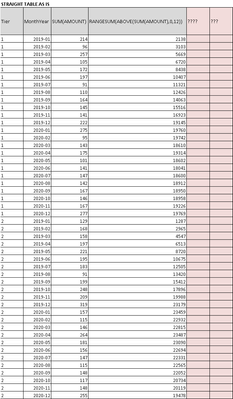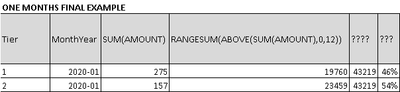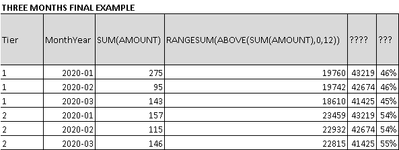Labels (1)
• ### Application Development

3 RepliesEmployeeContributor III
Author

Hi Miguel,

Thanks for the steer in the right direction, the following seems to work for a straight table If I sort by Tier

RangeSum(Above(Sum({<
Tier = {1,2,3,4,5}
>}[Euro_Equiv]), 0, 4))

/

RangeSum(Above(Sum({<
Tier = {1,2,3,4,5}
>}TOTAL <QuarterYear>[Euro_Equiv]), 0, 4))

Although when I convert it to a bar chart the sorting becomes an issue as the charts primary dimension is QuarterYear and not Tier. Do you know if  there a way to overide that as seems to only sort on the 1st Dimension (QuarterYear)?

As a straight Table (working as should)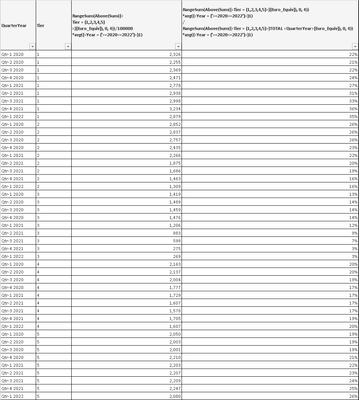As a chart not working due to sort on Quarter Year as Primary Dimension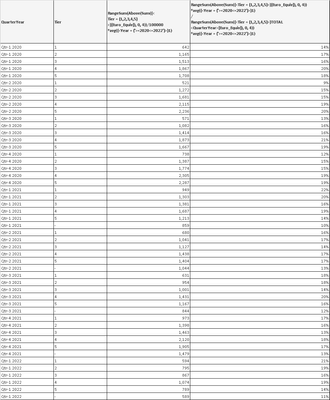Any help would be appreciated!

ThanksContributor III

The RangeSum() function in QlikView is used to do a selective sum on chosen fields which is not easily achieved by the sum function. It can take expressions containing other functions as its arguments and return the sum of those expressions.

One CognizantCommunity Browser